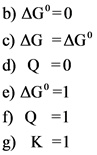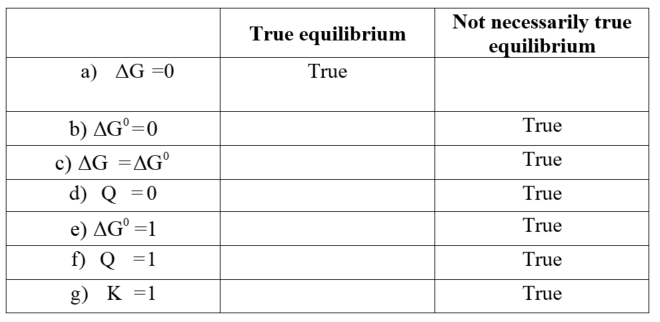# Which of the following conditions is always true at equilibrium

Which of the following conditions is always true at equilibrium.
So there are two boxes where I have to sort the choices in.
Box number 1 is called True at equilibrium. Box number 2 is called Not necessarily true at equilibrium.
Choices are
a)delta G=0
b)delta G^*=0
c)delta G = delta G^*
**d)Q=0 **
e)delta G^*=1
f)Q=1
g)K=1

Concepts and reason
A state in which the reactants and products have the same concentration with no change in time is represented as chemical equilibrium. In chemical equilibrium, the rate of the forward reaction is equal to the rate of the backward reaction.

Fundamentals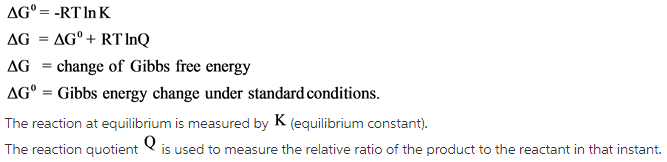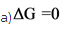The reaction at equilibrium represents the fact that there is no change in the concentration of the reactants and products, and the rate of both the forward and backward reactions are equal. In only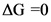, the equilibrium condition is satisfied. Therefore, the system will not work.